# 10.4: Electrophilic Additions to Alkenes

$$\newcommand{\vecs}{\overset { \rightharpoonup} {\mathbf{#1}} }$$ $$\newcommand{\vecd}{\overset{-\!-\!\rightharpoonup}{\vphantom{a}\smash {#1}}}$$$$\newcommand{\id}{\mathrm{id}}$$ $$\newcommand{\Span}{\mathrm{span}}$$ $$\newcommand{\kernel}{\mathrm{null}\,}$$ $$\newcommand{\range}{\mathrm{range}\,}$$ $$\newcommand{\RealPart}{\mathrm{Re}}$$ $$\newcommand{\ImaginaryPart}{\mathrm{Im}}$$ $$\newcommand{\Argument}{\mathrm{Arg}}$$ $$\newcommand{\norm}{\| #1 \|}$$ $$\newcommand{\inner}{\langle #1, #2 \rangle}$$ $$\newcommand{\Span}{\mathrm{span}}$$ $$\newcommand{\id}{\mathrm{id}}$$ $$\newcommand{\Span}{\mathrm{span}}$$ $$\newcommand{\kernel}{\mathrm{null}\,}$$ $$\newcommand{\range}{\mathrm{range}\,}$$ $$\newcommand{\RealPart}{\mathrm{Re}}$$ $$\newcommand{\ImaginaryPart}{\mathrm{Im}}$$ $$\newcommand{\Argument}{\mathrm{Arg}}$$ $$\newcommand{\norm}{\| #1 \|}$$ $$\newcommand{\inner}{\langle #1, #2 \rangle}$$ $$\newcommand{\Span}{\mathrm{span}}$$$$\newcommand{\AA}{\unicode[.8,0]{x212B}}$$

The reactions of alkanes discussed in Chapter 4 are homolytic processes, which means that the bonds are made and broken through radical or atomic intermediates. In contrast, the $$S_\text{N}$$ and $$E$$ reactions of alkyl halides, considered in Chapter 8, involve heterolytic bond cleavage and ionic reagents or products. An especially important factor contributing to the differences between the reactions of the alkanes and alkyl halides is the slight ionic character of $$\ce{C-H}$$ compared to $$\ce{C}$$-halide bonds (see Section 1-3). The alkenes are like the alkanes in being nonpolar compounds (Section 4-1) and it may come as a surprise that many important reactions of alkenes are heterolytic reactions. Why should this be so? No doubt because the electrons in the alkene double bonds are more exposed and accessible than the electrons in an alkane $$\ce{C-C}$$ bond.

This is evident from the atomic-orbital models of ethene described in Section 6-4C. The electrons of the double bond are pushed outward by their mutual repulsions, and their average positions are considerably farther from the bond axis than the electron positions of a single bond (Figure 10-6). In such circumstances, electrophilic reagents, which act to acquire electrons in chemical reactions (Section 8-1), are expected to be particularly reactive. This is actually the case. Furthermore, reagents that are primarily nucleophilic (electron-donating) are notoriously poor for initiating reactions at carbon-carbon double bonds. Exceptions occur when the double bonds carry substituents with a sufficiently high degree of electron-attracting power to reduce the electron density in the double bond enough to permit attack by a nucleophilic agent.Figure 10-6: Schematic representations of average densities of electrons in carbon-carbon single and double bonds.

Example of electrophilic reagents that normally add to carbon-carbon double bonds of alkenes to give saturated compounds include halogens ($$\ce{Cl_2}$$, $$\ce{Br_2}$$, and $$\ce{I_2}$$), hydrogen halides ($$\ce{HCl}$$ and $$\ce{HBr}$$), hypohalous acids ($$\ce{HOCl}$$ and $$\ce{HOBr}$$), water, and sulfuric acid:The mechanisms of these reactions have much in common and have been studied extensively from this point of view. They also have very considerable synthetic utility. The addition of water to alkenes (hydration) is particularly important for the preparation of a number of commercially important alcohols. Thus ethanol and 2-propanol (isopropyl alcohol) are made on a very large scale by the hydration of the corresponding alkenes (ethene and propene) using sulfuric or phosphoric acids as catalysts. The nature of this type of reaction will be described later.## The Stepwise Ionic Mechanism, Halogen Addition

We shall give particular attention here to the addition of bromine to alkenes because this reaction is carried out very conveniently in the laboratory and illustrates a number of important points about electrophilic addition reactions. Much of what follows applies to addition of the other halogens, except fluorine.

A significant observation concerning bromine addition is that it and many of the other reactions listed above proceed in the dark and are not influenced by radical inhibitors. This is evidence against a radical-chain mechanism of the type involved in the halogenation of alkanes (Section 4-4D). However, it does not preclude the operation of radical-addition reactions under other conditions, and, as we shall see later in this chapter, bromine, chlorine, and many other reagents that commonly add to alkenes by ionic mechanisms also can add by radical mechanisms.

One alternative to a radical-chain reaction for bromine addition to an alkene would be the simple four-center, one-step process shown in Figure 10-7.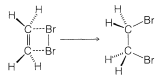Figure 10-7: Representation of a one-step suprafacial mechanism for addition of bromine to ethene. Gas-phase additions appear to proceed in this manner.

The mechanism of Figure 10-7 cannot be correct for bromine addition to alkenes in solution for two important reasons. First, notice that this mechanism requires that the two $$\ce{C-Br}$$ bonds be formed on the same side of the double bond, and hence produce suprafacial addition. However, there is much evidence to show that bromine and many other reagents add to alkenes to form antarafacial addition products (Figure 10-8).

Cyclohexene adds bromine to give trans-1,2-dibromocyclohexane:The cis isomer is not formed at all. To give the trans isomer, the two new $$\ce{C-Br}$$ bonds have to be formed on opposite sides of the double bond by antarafacial addition. But this is impossible by a one-step mechanism because the $$\ce{Br-Br}$$ bond would have to stretch too far to permit the formation of both $$\ce{C-Br}$$ bonds at the same time.

The second piece of evidence against the mechanism of Figure 10-7 is that bromine addition reactions carried out in the presence of more than one nucleophilic reagent usually give mixtures of products. Thus the addition of bromine to an alkene in methanol solution containing lithium chloride leads not only to the expected dibromoalkane, but also to products resulting from attack by chloride ions and by the solvent: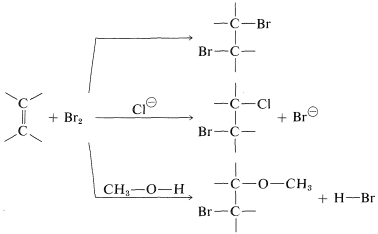The intervention of extraneous nucleophiles suggests a stepwise mechanism in which the nucleophiles compete for a reactive intermediate formed in one of the steps.

A somewhat oversimplified two-step mechanism that accounts for most of the foregoing facts is illustrated for the addition of bromine to ethene. [In the formation shown below, the curved arrows are not considered to have real mechanistic significance, but are used primarily to show which atoms can be regarded as nucleophilic (donate electrons) and which as electrophilic (accept electrons). The arrowheads always should be drawn to point to the atoms that are formulated as accepting a pair of electrons.]The first step (which involves electrophilic attack by bromine on the double bond) produces a bromide ion and a carbocation, as shown in Equation 10-1.$$^1$$

As we know from our study of $$S_\text{N}1$$ reactions (Section 8-4), carbocations react readily with nucleophilic reagents. Therefore in the second step of the bromine-addition mechanism, shown in Equation 10-2, the bromoethyl cation is expected to combine rapidly with bromide ion to give the dibromo compound. However, if other nucleophiles, such as $$\ce{Cl}^\ominus$$ or $$\ce{CH_3OH}$$, are present in solution, they should be able to compete with bromide ion for the cation, as in Equations 10-3 and 10-4, and mixtures of products will result:To account for the observation that all of these reactions result in antarafacial addition, we must conclude that the first and second steps take place from opposite sides of the double bond.

The simple carbocation intermediate of Equation 10-1 does not account for formation of the antarafacial-addition product. The results with $$S_\text{N}1$$ reactions (Section 8-6) and the atomic-orbital representation (see Section 6-4E) predict that the bonds to the positively charged carbon atom of a carbocation should lie in a plane. Therefore, in the second step of addition of bromine to cycloalkenes, bromide ion could attack either side of the planar positive carbon to give a mixture of cis- and trans-1,2-dibromocyclohexanes. Nonetheless, antarafacial addition occurs exclusively: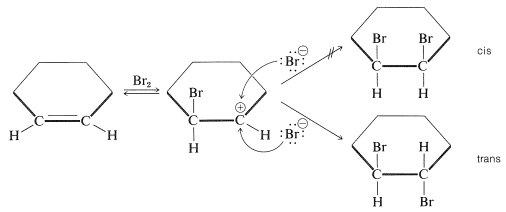To account for the stereospecificity of bromine addition to alkenes, it has been suggested that in the initial electrophilic attack of bromine a cyclic intermediate is formed that has bromine bonded to both carbons of the double bond. Such a "bridged" ion is called a bromonium ion because the bromine formally carries the positive charge:An $$S_\text{N}2$$-type of attack of bromide ion, or other nucleophile, at carbon on the side opposite to the bridging group then results in formation of the antarafacial-addition product: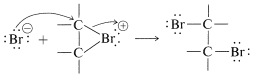We may seem to have contradicted ourselves because Equation 10-1 shows a carbocation to be formed in bromine addition, but Equation 10-5 suggests a bromonium ion. Actually, the formulation of intermediates in alkene addition reactions as "open" ions or as cyclic ions is a controversial matter, even after many years of study. Unfortunately, it is not possible to determine the structure of the intermediate ions by any direct physical method because, under the conditions of the reaction, the ions are so reactive that they form products more rapidly than they can be observed. However, it is possible to generate stable bromonium ions, as well as the corresponding chloronium and iodonium ions. The technique is to use low temperatures in the absence of any strong nucleophiles and to start with a 1,2-dihaloalkane and antimony pentafluoride in liquid sulfur dioxide: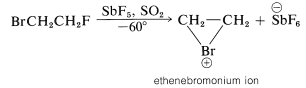The $$\ce{C_2H_4Br}^\oplus$$ ions produced in this way are relatively stable and have been shown by nmr to have the cyclic halonium ion structure.

## Complexes of Electrophilic Agents with Double Bonds

There is a further aspect of polar additions to alkenes that we should consider, namely, that electrophilic reagents form loose complexes with the $$\pi$$ electrons of the double bonds of alkenes prior to reaction by addition. Complexes of this type are called charge-transfer complexes (or $$\pi$$ complexes). Formation of a complex between iodine and cyclohexene is demonstrated by the fact that iodine dissolves in cyclohexene to give a brown solution, whereas its solutions in cyclohexane are violet. The brown solution of iodine in cyclohexene slowly fades as addition occurs to give colorless trans-1,2-diiodocyclohexane.

Precise Lewis structures cannot be written for charge-transfer complexes, but they commonly are represented aswith the arrow denoting that electrons of the double bond are associated with the electrophile. These complexes probably represent the first stage in the formation of addition products by a sequence such as the following for bromine addition:We have seen that electrophiles can react with alkenes to form carbon-halogen bonds by donating positive halogen, $$\ce{Br}^\oplus$$, $$\ce{Cl}^\oplus$$, or $$\ce{I}^\oplus$$. Likewise, carbon-hydrogen bonds can be formed by appropriately strong proton donors, which, of course, are typically strong proton acids. These acids are more effective in the absence of large amounts of water because water can compete with the alkene as a proton acceptor (also see Section 10-3E). Hydrogen chloride addition to ethene occurs by way of a proton-transfer step to give the ethyl cation and a chloride ion (Equation 10-6) followed by a step in which the nucleophilic chloride ion combines with the ethyl cation (Equation 10-7):All of the hydrogen halides $$\ce{HF}$$, $$\ce{HCl}$$, $$\ce{HBr}$$, and $$\ce{HI}$$) will add to alkenes. Addition of hydrogen fluoride, while facile, is easily reversible. However, a solution of $$70\%$$ anhydrous hydrogen fluoride and $$30\%$$ of the weak organic base, pyridine, which is about 1/10,000 times as strong as ammonia, works better, and with cyclohexene gives fluorocyclohexane. With hydrogen iodide, care must be taken to prevent $$\ce{I_2}$$ addition products resulting from iodine formed by oxidation reactions such as

$4 \ce{HI} + \ce{O_2} \rightarrow 2 \ce{I_2} + 2 \ce{H_2O}$

With hydrogen bromide, radical-chain addition may intervene unless the reaction conditions are controlled carefully (this will be discussed in Section 10-7).

The stereochemistry of addition depends largely on the structure of the alkene, but for simple alkenes and cycloalkenes, addition occurs predominantly in an antarafacial manner. For example, hydrogen bromide reacts with 1,2-dimethylcyclohexene to give the antarafacial addition product: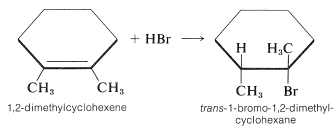## Hydration

We mentioned previously that the hydration of alkenes required a strong acid as a catalyst, because water itself is too weak an acid to initiate the proton-transfer step. However, if a small amount of a strong acid such as sulfuric acid is present, hydronium ions, $$\ce{H_3O}^\oplus$$, are formed in sufficient amount to protonate reasonably reactive alkenes, although by no means as effectively as does concentrated sulfuric acid. The carbocation formed then is attacked rapidly by a nucleophilic water molecule to give the alcohol as its conjugate acid,$$^2$$ which regenerates hydronium ion by transferring a proton to water. The reaction sequence follows for 2-methylpropene: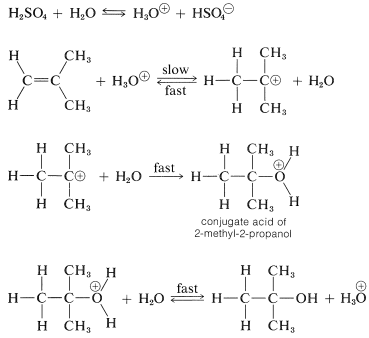In this sequence, the acid acts as a catalyst because the hydronium ion used in the proton addition step is regenerated in the final step.

Sulfuric acid (or phosphoric acid) is preferred as an acid catalyst for addition of water to alkenes because the conjugate base, $$\ce{HSO_4-}$$ (or $$\ce{H_2PO_4-}$$), is a poor nucleophile and does not interfere in the reaction. However, if the water concentration is kept low by using concentrated acid, addition occurs to give sulfate (or phosphate) esters. The esters formed with sulfuric acid are either alkyl acid sulfates $$\ce{R-OSO_3H}$$ or dialkyl sulfates $$\ce{(RO)_2SO_2}$$. In fact, this is one of the major routes used in the commercial production of ethanol and 2-propanol. Ethen and sulfuric acid give ethyl hydrogen sulfate, which reacts readily with water in a second step to give ethanol:## Aqueous versus Nonaqueous Acids. Acid Strengths

One of the more confusing features of organic chemistry is the multitude of conditions that are used to carry out a given kind of reaction, such as the electrophilic addition of proton acids to different alkenes. Strong acids, weak acids, water, no water - Why can't there be a standard procedure? The problem is that alkenes have very different tendencies to accept protons. In the vapor phase, $$\Delta H^0$$ for addition of a proton to ethene is about $$35 \: \text{kcal}$$ more positive than for 2-methylpropene, and although the difference should be smaller in solution, it still would be large. Therefore we can anticipate (and we find) that a much more powerful proton donor is needed to initiate addition of an acid to ethene than to 2-methylpropene. But why not use in all cases a strong enough acid to protonate any alkene one might want to have a proton acid add to? Two reasons: First, strong acids can induce undesirable side reactions, so that one usually will try not to use a stronger acid than necessary; second, very strong acid may even prevent the desired reaction from occurring!

In elementary chemistry, we usually deal with acids in more or less dilute aqueous solution and we think of sulfuric, hydrochloric, and nitric acids as being similarly strong because each is essentially completely disassociated in dilute water solution:

$\ce{HCl} + \ce{H_2O} \overset{\longrightarrow}{\leftarrow} \ce{H_3O}^\oplus + \ce{Cl}^\ominus$

This does not mean they actually are equally strong acids. It means only that each of the acids is sufficiently strong to donate all of its protons to water. We can say that water has a "leveling effect" on acid strengths because as long as an acid can donate its protons to water, the solution has but one acid "strength" that is determined by the $$\ce{H_3O}^\oplus$$ concentration, because $$\ce{H_3O}^\oplus$$ is where the protons are.

Now, if we use poorer proton acceptors as solvent we find the proton-donating powers of various "strong" acids begin to spread out immensely. Furthermore, new things begin to happen. For example, ethene is not hydrated appreciably by dilute aqueous acid; it just is too hard to transfer a proton from hydronium ion to ethene. So we use concentrated sulfuric acid, which is strong enough to add a proton to ethene. But now we don't get hydration, because any water that is present in concentrated sulfuric acid is virtually all converted to $$\ce{H_3O}^\oplus$$, which is non-nucleophilic!

$\ce{H_2SO_4} + \ce{H_2O} \rightarrow \ce{H_3O}^\oplus + \ce{HSO_4-}$

However, formation of $$\ce{H_3O}^\oplus$$ leads to formation of $$\ce{HSO_4-}$$, which has enough nucleophilic character to react with the $$\ce{CH_3CH_2+}$$ to give ethyl hydrogen sulfate and this is formed instead of the conjugate acid of ethanol (Section 10-3E). The epitome of the use of stronger acid and weaker nucleophile is with liquid $$\ce{SO_2}$$ (bp $$\sim 10^\text{o}$$) as the solvent and $$\ce{HBF_6}$$ as the acid. This solvent is a very poor proton acceptor (which means that its conjugate acid is a very good proton donor) and $$\ce{SbF_6-}$$ is an extremely poor nucleophile. If we add ethene to such a solution, a stable solution of $$\ce{CH_3CH_2+} \ce{SbF_6-}$$ is formed. The reason is that there is no better proton acceptor present than $$\ce{CH_2=CH_2}$$ and no nucleophile good enough to combine with the cation.

## A Biological Hydration Reaction

The conversion of fumaric acid to malic acid is an important biological hydration reaction. It is one of a cycle of reactions (Krebs citric acid cycle) involved in the metabolic combustion of fuels (amino acids and carbohydrates) to $$\ce{CO_2}$$ and $$\ce{H_2O}$$ in a living cell.Figure 10-9.Figure 10-9: Representation of the course of enzyme-induced hydration of fumaric acid (trans-butenedioic acid) to give L-malic acid (L-2-hydroxy-butanedioic acid). If the enzyme complexes with either $$\ce{-CO_2H}$$ (carboxyl) group of fumaric acid, and then adds $$\ce{OH}$$ from its right hand and $$\ce{H}$$ from its left, the proper stereoisomer (L) is produced by antarafacial addition to the double bond. At least three particular points of contact must occur between enzyme and substrate to provide the observed stereospecificity of the addition. Thus, if the enzyme functions equally well with the alkenic hydrogen or the carboxyl toward its mouth (as shown in the drawing) the reaction still will give antarafacial addition, but D,L-malic acid will be the product.

$$^1$$An alternative to Equation 10-1 would be to have $$\ce{Br_2}$$ ionize to $$\ce{Br}^\oplus$$ and $$\ce{Br}^\ominus$$, with a subsequent attack of $$\ce{Br}^\oplus$$ on the double bond to produce the carbocation. The fact is that energy required for such an ionization of $$\ce{Br_2}$$ is prohibitively large even in water solution $$\left( \Delta H^0 \geq 80 \: \text{kcal} \right)$$. One might well wonder why Equation 10-1 could possibly be more favorable. The calculated $$\Delta H^0$$ for $$\ce{CH_2=CH_2} + \ce{Br_2} \rightarrow \cdot \ce{CH_2-CH_2Br} + \ce{Br} \cdot$$ is $$+41 \: \text{kcal}$$, which is only slightly more favorable than the $$\Delta H^0$$ for $$\ce{Br_2} \rightarrow 2 \ce{Br} \cdot$$ of $$46.4 \: \text{kcal}$$. However, available thermochemical data suggest that the ease of transferring an electron from $$\cdot \ce{CH_2CH_2Br}$$ to $$\ce{Br} \cdot$$ to give $$^\oplus \ce{CH_2CH_2Br} + \ce{Br}^\ominus$$ is about $$80 \: \text{kcal}$$ more favorable than $$2 \ce{Br} \cdot \rightarrow \ce{Br}^\oplus + \ce{Br}^\ominus$$. Thus the overall $$\Delta H^0$$ of Equation 10-1 is likely to be about $$85 \: \text{kcal}$$ more favorable than $$\ce{Br_2} \rightarrow \ce{Br}^\oplus + \ce{Br}^\ominus$$.

$$^2$$The terms conjugate acid and conjugate base are very convenient to designate substances that are difficult to name simply as acids, bases, or salts. The conjugate acid of a compound $$\ce{X}$$ is $$\ce{XH}^\oplus$$ and the conjugate base of $$\ce{HY}$$ is $$\ce{Y}^\ominus$$. Thus $$\ce{H_3O}^\oplus$$ is the conjugate acid of water, while $$\ce{OH}^\ominus$$ is its conjugate base. Water itself is then both the conjugate base of $$\ce{H_3O}^\oplus$$ and the conjugate acid of $$\ce{OH}^\ominus$$

## References

John D. Robert and Marjorie C. Caserio (1977) Basic Principles of Organic Chemistry, second edition. W. A. Benjamin, Inc. , Menlo Park, CA. ISBN 0-8053-8329-8. This content is copyrighted under the following conditions, "You are granted permission for individual, educational, research and non-commercial reproduction, distribution, display and performance of this work in any format."